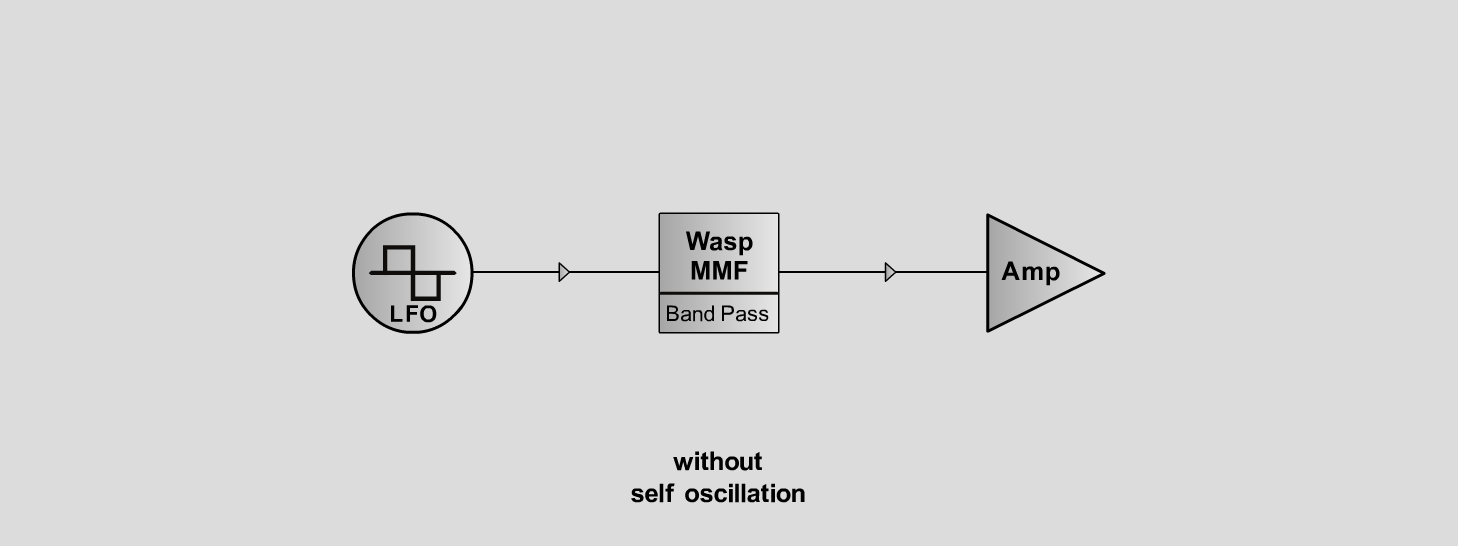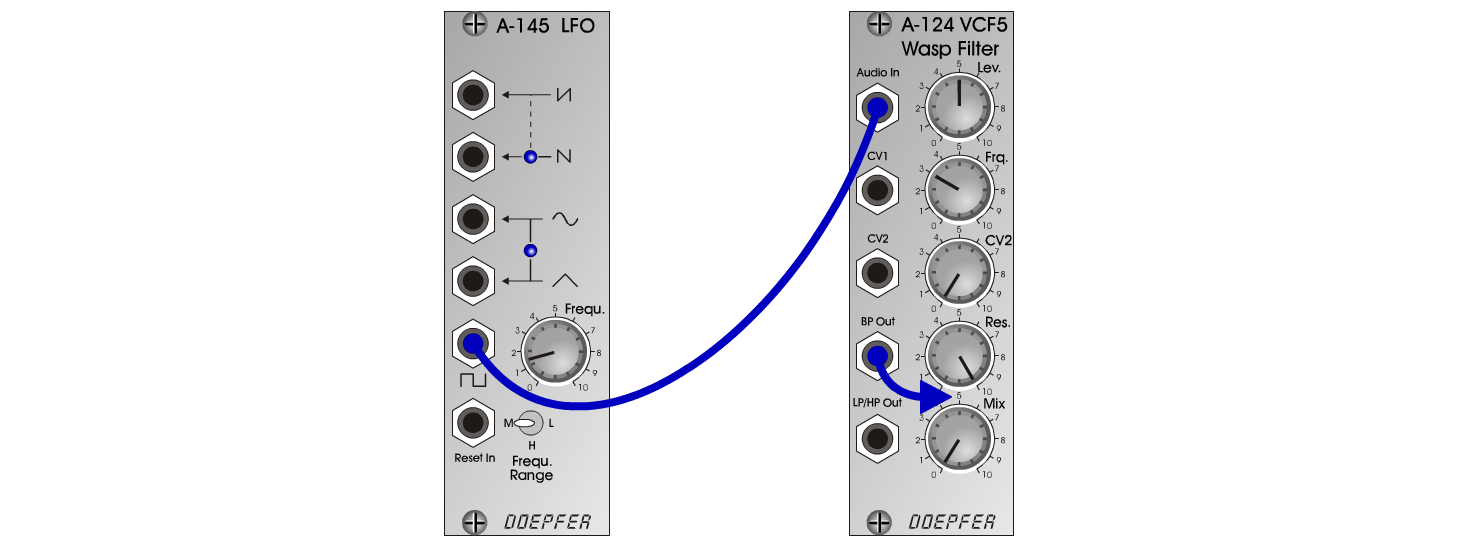Wasp-DrumD r u m sD o e p f e r A - 100 Connections: Settings: Wasp-Drum 1 A-145 (Square) <=> A-124 (Audio In)  A-124 (BP Out) <=> Amp A-124 (Lev = 5, Frq = 3, CV 2 = 0, Res = 10, Mix = 0)  A-145 (Frq = 1.5, Range = M) Wasp-Drum 2 A-124 (Lev = 5, Frq = 3, CV 2 = 0, Res = 10, Mix = 0)  A-145 (Frq = 7.5, Range = M) Wasp-Drum 3 A-124 (Lev = 5, Frq = 3.2, CV 2 = 0, Res = 9, Mix = 0)  A-145 (Frq = 1.5, Range = M) Wasp-Drum 4 A-124 (Lev = 5, Frq = 3.5, CV 2 = 0, Res = 10, Mix = 0)  A-145 (Frq = 1.5, Range = M) Wasp-Drum 5 A-124 (Lev = 5, Frq = 3.5, CV 2 = 0, Res = 8.5, Mix = 0)  A-145 (Frq = 1.5, Range = M) Wasp-Drum 6 A-124 (Lev = 7, Frq = 3.7, CV 2 = 0, Res = 8.5, Mix = 0)  A-145 (Frq = 1.5, Range = M) Wasp-Drum 7 A-124 (Lev = 7, Frq = 3.9, CV 2 = 0, Res = 8.5, Mix = 0)  A-145 (Frq = 1.5, Range = M) Wasp-Drum 8 A-124 (Lev = 7, Frq = 4, CV 2 = 0, Res = 8.5, Mix = 0)  A-145 (Frq = 1.5, Range = M) Wasp-Drum 9 A-124 (Lev = 7, Frq = 4.2, CV 2 = 0, Res = 8.5, Mix = 0)  A-145 (Frq = 1.5, Range = M) Wasp-Drum 10 A-124 (Lev = 7, Frq = 4.2, CV 2 = 0, Res = 9, Mix = 0)  A-145 (Frq = 1.5, Range = M) Wasp-Drum 11 A-124 (Lev = 5, Frq = 4.5, CV 2 = 0, Res = 8.5, Mix = 0)  A-145 (Frq = 1.5, Range = M) Wasp-Drum 12 A-124 (Lev = 5, Frq = 4.8, CV 2 = 0, Res = 10, Mix = 0)  A-145 (Frq = 1.5, Range = M) Wasp-Drum 13 A-124 (Lev = 5, Frq = 5, CV 2 = 0, Res = 10, Mix = 0)  A-145 (Frq = 1.5, Range = M) Wasp-Drum 14 A-124 (Lev = 5.2, Frq = 3, CV 2 = 0, Res = 10, Mix = 0)  A-145 (Frq = 1.5, Range = M) Wasp-Drum 15 A-124 (Lev = 5.6, Frq = 3, CV 2 = 0, Res = 10, Mix = 0)  A-145 (Frq = 1.5, Range = M) Josef MuellerSound samples Wasp-Drum Piece (arr. by Marvin Mueller) Wasp-Drum 1 Wasp-Drum 2 Wasp-Drum 3 Wasp-Drum 4 Wasp-Drum 5 Wasp-Drum 6 Wasp-Drum 7 Wasp-Drum 8 Wasp-Drum 9 Wasp-Drum 10 Wasp-Drum 11 Wasp-Drum 12 Wasp-Drum 13 Wasp-Drum 14 Wasp-Drum 15